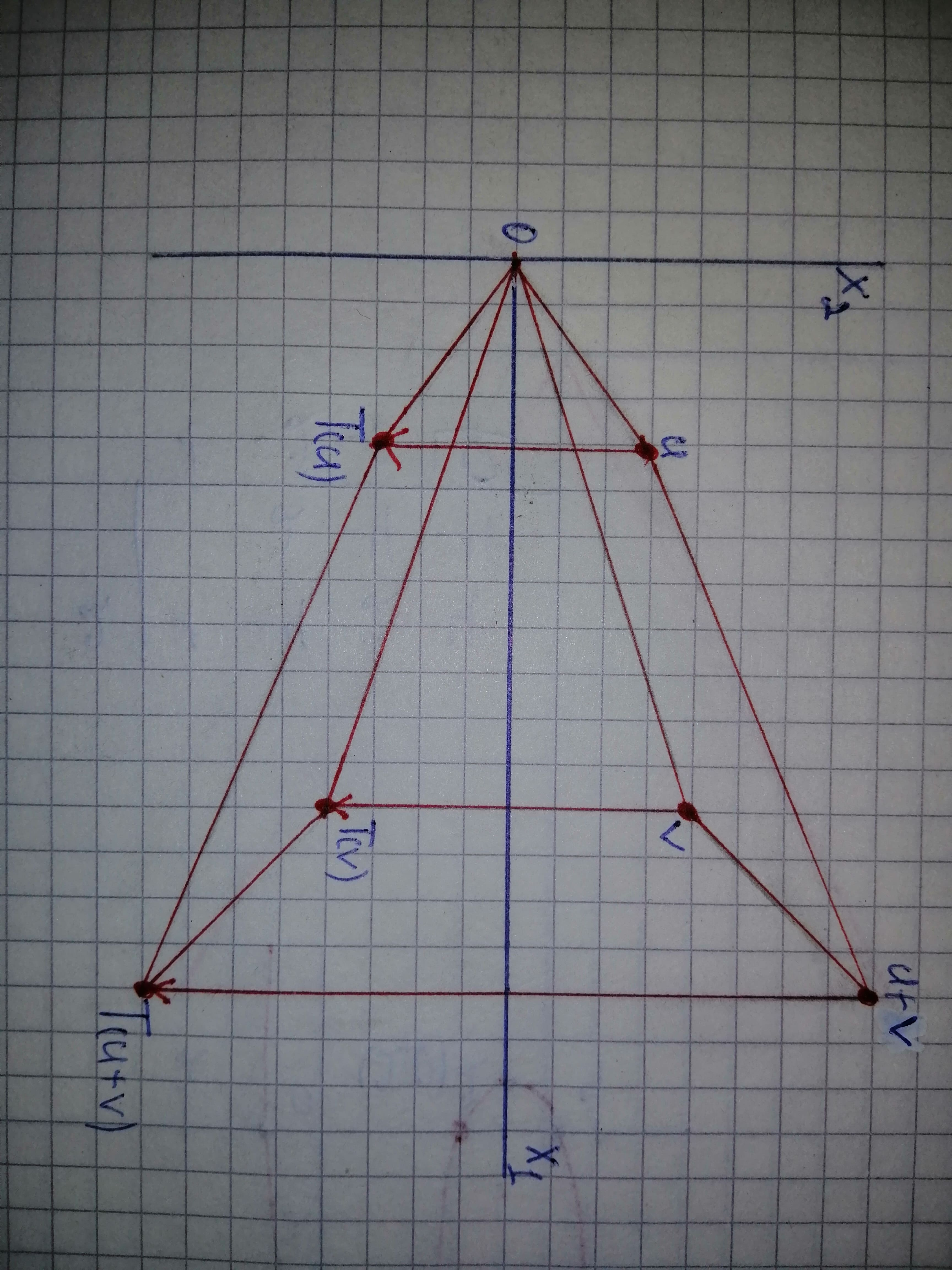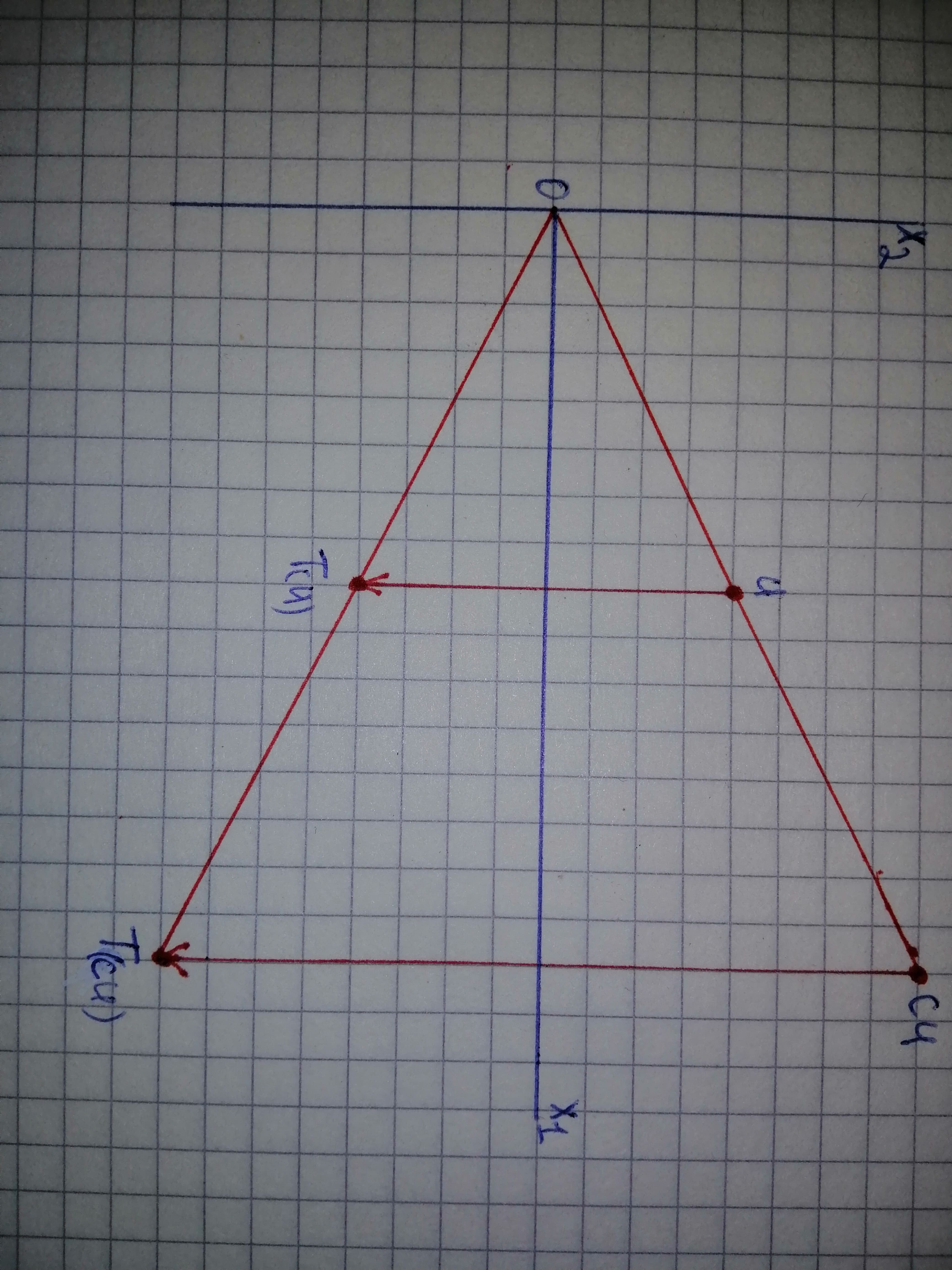# To sketch: (i) The properties, (ii) Linear transformation. Let {T}:mathbb{R}^{2}tomathbb{R}^{2} be the linear transformation that reflects each point through the x_{1} axis. Let {A}={left[begin{matrix}{1}&{0}{0}&-{1}end{matrix}right]}preprekomW 2021-02-03 Answered
To sketch:
(i) The properties,
(ii) Linear transformation.
Let $T:{\mathbb{R}}^{2}\to {\mathbb{R}}^{2}$ be the linear transformation that reflects each point through the
${x}_{1}axis.$
Let $A=\left[\begin{array}{cc}1& 0\\ 0& -1\end{array}\right]$
You can still ask an expert for help

• Questions are typically answered in as fast as 30 minutes

Solve your problem for the price of one coffee

• Math expert for every subject
• Pay only if we can solve itwheezym

Assume the value of $u=\left[\begin{array}{c}2\\ 4\end{array}\right],v=\left[\begin{array}{c}6\\ 3\end{array}\right].$
Consider some random points for u and v.
The value of
$u+v=\left[\begin{array}{c}2\\ 4\end{array}\right]+\left[\begin{array}{c}6\\ 3\end{array}\right]$
$=\left[\begin{array}{c}8\\ 7\end{array}\right]$
The transformation -axis.
Determine the value of $T\left(u\right):$
$T\left(u\right)=Au$
$=\left[\begin{array}{cc}1& 0\\ 0& -1\end{array}\right]\left[\begin{array}{c}2\\ 4\end{array}\right]$
$=\left[\begin{array}{c}2\\ -4\end{array}\right]$
Determine the value of $T\left(v\right):$
$T\left(v\right)=Av$
$=\left[\begin{array}{cc}1& 0\\ 0& -1\end{array}\right]\left[\begin{array}{c}6\\ 3\end{array}\right]$
$=\left[\begin{array}{c}6\\ -3\end{array}\right]$
Determine the value of

$=\left[\begin{array}{cc}1& 0\\ 0& -1\end{array}\right]\left[\begin{array}{c}8\\ 7\end{array}\right]$
$=\left[\begin{array}{c}8\\ -7\end{array}\right]$
Show the values of the vectors as in Figure 1.Linear transformation:
Consider the value of c as 2.
Thus the value of cu is:
$cu=2×\left[\begin{array}{c}2\\ 4\end{array}\right]=\left[\begin{array}{c}4\\ 8\end{array}\right]$
Determine the transformation of cu.
$T\left(cu\right)=\left[\begin{array}{cc}1& 0\\ 0& -1\end{array}\right]\left[\begin{array}{c}4\\ 8\end{array}\right]$
$=\left[\begin{array}{c}4\\ -8\end{array}\right]$
Show the linear transformation of T as in Figure 2.